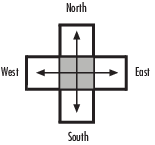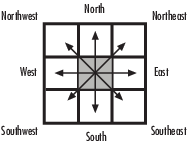Documentation

Trace Boundary

Trace object boundaries in binary images

Library

Analysis & Enhancement

visionanalysisDescription

The Trace Boundary block traces object boundaries in binary images, where nonzero pixels represent objects and 0 pixels represent the background.

Port Descriptions

PortInput/OutputSupported Data Types

BW

Vector or matrix that represents a binary image

Boolean

Start Pt

One-based [x y] coordinates of the boundary starting point.

• Double-precision floating point

• Single-precision floating point

• 8-, 16-, and 32-bit signed integer

• 8-, 16-, and 32-bit unsigned integer

Pts

M-by-2 matrix of [x y] coordinates of the boundary points, where M represents the number of traced boundary pixels. M must be less than or equal to the value specified by the Maximum number of boundary pixels parameter.

$\left[\begin{array}{cc}{x}_{1}& {y}_{1}\\ {x}_{2}& {y}_{2}\\ {x}_{3}& {y}_{3}\\ .& .\\ .& .\\ .& .\\ {x}_{m}& {y}_{m}\end{array}\right]$

Same as Start Pts port

Parameters

Connectivity

Specify which pixels are connected to each other. If you want a pixel to be connected to the pixels on the top, bottom, left, and right, select 4. If you want a pixel to be connected to the pixels on the top, bottom, left, right, and diagonally, select 8. For more information about this parameter, see the Label block reference page.

Initial search direction

Specify the first direction in which to look to find the next boundary pixel that is connected to the starting pixel.

If, for the Connectivity parameter, you select 4, the following figure illustrates the four possible initial search directions:If, for the Connectivity parameter, you select 8, the following figure illustrates the eight possible initial search directions:Trace direction

Specify the direction in which to trace the boundary. Your choices are Clockwise or Counterclockwise.

Maximum number of boundary pixels

Specify the maximum number of boundary pixels for each starting point. The block uses this value to preallocate the number of rows of the Pts port output matrix so that it can hold all the boundary pixel location values.

Use the Maximum number of boundary pixels parameter to specify the maximum number of boundary pixels for the starting point.